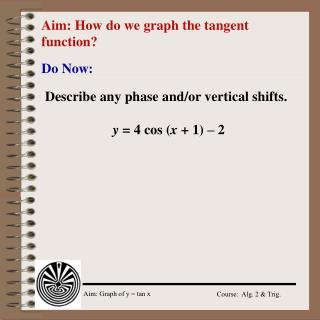Download PresentationAim: How do we graph the tangent function?

# Aim: How do we graph the tangent function? - PowerPoint PPT PresentationDownload Presentation## Aim: How do we graph the tangent function?

- - - - - - - - - - - - - - - - - - - - - - - - - - - E N D - - - - - - - - - - - - - - - - - - - - - - - - - - -
##### Presentation Transcript

1. Aim: How do we graph the tangent function? Do Now: Describe any phase and/or vertical shifts. y = 4 cos (x + 1) – 2

2. Model Problem Describe any phase and/or vertical shifts. y = .5 sin 3(x - ) – /3 Write an equation for each transformation. y = sin x; /2 units to right and 3.5 units up

3. Regents Prep Which function is a translation of y = sin  that is /3 units up and /2 units to the left? What is the period of the function

4. 2 1, tan π/3 1, tan π/4 1 1, tan π/6 π 1, tan -(π/4) -1 1, tan -(π/3) -2 tan 0/1 = 0 Tangent and the Unit Circle unit circle 1 A line tangent to a circle intersects the circle in exactly one point.

5. π vertical asymptotes Asymptotes of the Tangent Curve y = tan  2 tangent function is discontinuous x = π/2 1 -1 y = tan (π/2) = ? UND x = -π/2 y = tan (-π/2) = ? UND -2 y = tan (-3π/2) = ? UND

6. The a factor: Because y, in the tangent function extends to positive and negative infinity, there is no amplitude. The asymptotes remain the same (if b = 1). Only the shape of the curve changes.As a gets bigger, the curve becomes straighter. The bfactor: The period of the tangent curve y = tan is π. The period of the tangent curve y = tan b is The a and b of the Tangent Curve y = atan b

7. π -π The Tangent Curve y = atan b 2 x = π/2 1 -1 vertical asymptotes at the end of each cycle x = -π/2 -2 Key Points for graphing y = tan  : asymptotes: -π/2 and π/2; and -π/4, 0, π/4.

8. tan 0 = 0 when y = ±1 Model Problem Sketch the graph y = tan 1/2  from 0 to π On same axes, graph y = tan (-1/2) asymptote  = -π asymptote  = π 1 -π π -1

9. Model Problem An architect is designing the front façade of a building to include a triangle, as shown in the figure. The base of the triangle is 200 ft. wide. The function y = 100 tan  models the height of the triangle, where  is the angle indicated. Graph the function in degree modes. What is the height of the triangle when  = 16o? What is the height when  = 22o?  200’

10. Regents Prep Which pair of values are NOT equal? Which function has a period of 4?